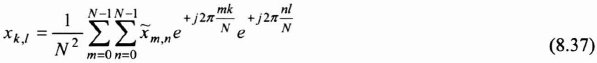## 8.5. Filtering in the frequency domain

When the filter properties are specified in the frequency domain, or when the impulse responses have a very large support, it is advantageous to carry out the filtering operation in the Fourier domain by using equation (8.5). Changing the representation domain is done using the 2-D discrete Fourier transform. Consequently, the input/output relation, in the spatial domain, is no longer described by a simple discrete convolution equation, but by a circular convolution equation. We will look at the effects of this phenomenon at the end of this section.

### 8.5.1. 2-D discrete Fourier transform (DFT)

Let us look at a sequence of two indices {xk,l}. To simplify our presentation, we assume that the two indices have the same variation domain, from 0 to N−1. The transformed sequenceis given by:It is clear that the transform sequence is periodic, of period N for each index.

Equation (8.36) also shows that the transformation is separable, being constituted of a discrete 1-D Fourier transform (DFT) operating row by row, followed with a DFT column by column.

As in the 1-D context, the 2-D discrete Fourier transform is inversible:As in the 1-D context, we ...

Get Digital Filters Design for Signal and Image Processing now with the O’Reilly learning platform.

O’Reilly members experience live online training, plus books, videos, and digital content from nearly 200 publishers.